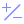#RandomDouble (PQL)

Returns a random decimal number between the lower and upper bounds provided.

• Returned Output: Numeric
• Library: PQL \ Common \ Math
• Version: 2018.00.000
• Compatibility:
• Can be combined with and other PQL function throughout the application.
• It CANNOT be used with MDX or VBA functions. But it can be used on MDX-based content in other parts of the application.

#### Syntax

RandomDouble( <Numeric> , <Numeric> )

* Click on the function's arguments above for more details on the input values.

• The first numeric argument is the lower bound; the second is the upper bound.
• The bound values can be any number.
##### Different Function types
• This function is like the Excel random function.
• Also see Random for random integer numbers.

#### Examples

This example randomly returns "0.251" , picking a number between 0 and 1.

RandomDouble(0,1)

This example randomly returns -0.13 , picking a number between -1 and 1.

RandomDouble(-1,1)